• 描述一个函数isPrime(n)用于判断一个数字n是不是素数，用户输入一个正整数，在一行内输出不大于该数的所有素数，各数后面用一个空格分隔。输入格式输入一个正整数输出格式不大于该数的所有素数，各数后面用一个...


描述写一个函数isPrime(n)用于判断一个数字n是不是素数，用户输入一个正整数，在一行内输出不大于该数的所有素数，各数后面用一个空格分隔。输入格式输入一个正整数输出格式不大于该数的所有素数，各数后面用一个空格分隔。输入输出示例输入输出示例 11002 3 5 7 11 13 17 19 23 29 31 37 41 43 47 53 59 61 67 71 73 79 83 89 97  解析：    素数的概念又称为质数，一个大于1的自然数，除了1和它自身外，不能被其他自然数整除的数。    可以定义一个函数，传入一个整数，返回其是否为素数。判定一个数是否为素数的方法是，用传入的整数 n 除从2到n-1之间的每一个整数，如果存在某个可以整除的数，则其不是素数，如果没有任何除1和其本身以外的因子，则其是素数。(时间复杂度为o(n))# 将判断一个整数 n 是否为素数的代码定义为一个函数isPrime(n)# 传入参数为整数 n，n 是素数时返回值为True，否则返回False# 时间复杂度为o(n)def isPrime(n):  # 判断参数 n 是否为素数的函数    if n <= 1:   # 小于2的数字都不是素数        return False    for i in range(2,n):   # 根据素数定义判定是否是素数，是素数返回1        if n % i == 0:     # 从 2到n-1中如果存在一个数是i，使n 可以整除i，则n不是素数            return False    else:                  # 若for循环未遇到return正常结束，则n是素数        return True# 以下代码调用定义的isPrime()判定num是否为素数，输出小于m的所有素数m = int(input())               # 输入一个正整数for num in range(m):           # 获得小于m的整数数列    if isPrime(num):           # 如果isPrime(num)返回值为True,num 是素数        print(num,end = ' ')   # 输出num# 输入 100# 输出 2 3 5 7 11 13 17 19 23 29 31 37 41 43 47 53 59 61 67 71 73 79 83 89 97    其主程序部分可以修改为以下模式，使当前文件可以做为模块被其他程序调用。当文件直接被运行时，if __name__ == "__main__":语句后的语句块中的输入输出等语句会被执行。当文件被当做模块import时，只调用其中的函数，if __name__ == "__main__":后的语句块不会被执行。# 将判断一个整数 n 是否为素数的代码定义为一个函数isPrime(n)# 传入参数为整数 n，n 是素数时返回值为True，否则返回False# 时间复杂度为o(n)def isPrime(n):  # 判断参数 n 是否为素数的函数    if n <= 1:   # 小于2的数字都不是素数        return False    for i in range(2,n):   # 根据素数定义判定是否是素数，是素数返回1        if n % i == 0:     # 从 2到n-1中如果存在一个数是i，使n 可以整除i，则n不是素数            return False    else:                  # 若for循环未遇到return正常结束，则n是素数        return True# __name__ 是当前模块名，当模块被直接运行时模块名为 __main__ 。# 当模块被直接运行时，以下代码块将被运行，当模块是被导入时，代码块不被运行。# 可以删除if __name__ == "__main__":，将下面语句块向前提一级# 以下代码调用定义的isPrime()判定num是否为素数，输出小于m的所有素数if __name__ == "__main__":    m = int(input())       # 输入一个正整数    for num in range(m):   # 获得小于m的整数数列        if isPrime(num):   # 如果isPrime(num)返回值为True,num 是素数            print(num,end = ' ')     # 输出num# 输入 100# 输出 2 3 5 7 11 13 17 19 23 29 31 37 41 43 47 53 59 61 67 71 73 79 83 89 97    我们知道一个数若可以进行因数分解，那么分解时得到的两个数一定是一个小于等于sqrt(n)，一个大于等于sqrt(n)，所以对于每个数n，并不需要从2判断到n-1，遍历到sqrt(n)即可。因为若sqrt(n)左侧找不到约数，那么右侧也一定找不到约数。这样可以显著提升算法的效率，上面判断素数函数可以修改为：# 传入参数为整数 n，n 是素数时返回值为True，否则返回False# 时间复杂度为o(n)def isPrime(n):  # 判断参数 n 是否为素数的函数    if n <= 1:   # 小于2的数字都不是素数        return False    for i in range(2,n):   # 根据素数定义判定是否是素数，是素数返回1        if n % i == 0:     # 从 2到n-1中如果存在一个数是i，使n 可以整除i，则n不是素数            return False    else:                  # 若for循环未遇到return正常结束，则n是素数        return True   依据经验，我们知道素数分布的规律，除了2以外的所有素数都是奇数，所以可以只判定奇数是否是素数就可以，可以减少一半的判定素数的计算量。if __name__ == "__main__":    m = int(input())       # 输入一个正整数    if m > 2:        print(2,end = ' ')    for num in range(3,m,2): # 获得小于m的整数数列        if isPrime(num):     # 如果isPrime(num)返回值为True,num 是素数            print(num,end = ' ')     # 输出num    素数分布的规律：大于等于5的素数一定和6的倍数相邻。例如5和7，11和13,17和19等等。查看100以内的素数：2 3   5 7    11 13    17 19    23    29 31    37    41 43    47 53    59 61   67    71 73    79 83 89 97    可以发现除2和3外，其他数都是6的倍数的相邻数：6-1，6+1，2*6-1，2*6+1，3*6-1，3*6+1...    但要注意，6 的倍数的相邻数并不全是素数，例如35。所以还是需要调用素数判定函数进行判定。只判定6的倍数的相邻数是否是素数就可以找到所有素数。这样可以进一步减少判定的次数，提高效率。注意2和3要单独判定。if __name__ == "__main__":    m = int(input())       # 输入一个正整数    if m > 2:        print(2,end = ' ')    if m > 3:        print(3,end = ' ')    for num in range(0,m,6):   # 获得小于m的整数数列        if isPrime(num - 1):   # 如果isPrime(num -1)返回值为True,num 是素数            print(num - 1,end = ' ')     # 输出num - 1        if isPrime(num + 1):   # 如果isPrime(num + 1)返回值为True,num 是素数            print(num + 1,end = ' ')     # 输出num + 1       综合以上分析，可以同时优先判定素数的函数和判定的代码：def isPrime(n):  # 判断参数 n 是否为素数的函数    if n <= 1:      # 小于2的数字都不是素数        return False    for i in range(2,int(n ** 0.5) + 1):   # 两因子相同时，为保证取到右边界，需加1    # for i in range(2,int(math.sqrt(n)) + 1)        if n % i == 0:     # 从 2到n-1中如果存在一个数是i，使n 可以整除i，则n不是素数            return False    else:                  # 若for循环未遇到return正常结束，则n是素数        return Trueif __name__ == "__main__":    m = int(input())       # 输入一个正整数    if m > 2:        print(2,end = ' ')    if m > 3:        print(3,end = ' ')    for num in range(0,m,6):   # 获得小于m的整数数列        if isPrime(num - 1):   # 如果isPrime(num -1)返回值为True,num 是素数            print(num - 1,end = ' ')     # 输出num - 1        if isPrime(num + 1):   # 如果isPrime(num + 1)返回值为True,num 是素数            print(num + 1,end = ' ')     # 输出num + 1        除了这些方法以外，还可以使用筛选法，先生成小于n的所有数字，再把 2 到 sqrt(n) 之间的整数的倍数依次去掉，剩余的就是小于n的所有素数了。这种方法的效率更高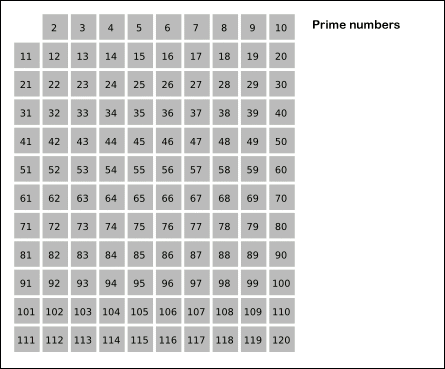(注：此图片来自网络，版权归原作者所有，此处仅用于教学演示)def isprime(n):  '''筛选法获取小于n的所有素数'''  ls = list(range(3, n, 2))          # 待判断整数  for index in range(int(n ** 0.5)): # 最大整数的平方根    current = ls[index]    if current > int(n ** 0.5): # 如果当前数字已大于最大整数的平方根，结束判断      break    # 对该位置之后的元素进行过滤    ls[index + 1:] = list(filter(lambda x: 0 if not x%current else x,ls[index + 1:]))  return  + ls   # 2是素数，单独加上去# 输出100以内所有素数 print(*isprime(100))


展开全文• 输出的幅值跟正常的对上 下附波形输出 ![图片说明](https://img-ask.csdn.net/upload/202005/15/1589502914_1887.jpg) 系统框图如下： ![图片说明]...测试用例 测试用例
• 在找一段信号的波峰波谷的过程中，除了可以用matlab中自带的findpeaks函数，这里给出自己的找波峰的函数 function [k,v]=findpeaks(x,m,w) 输入： 1 只有一个输入x，表示要找到峰值的信号； 2 m，表示模式，有...
在找一段信号的波峰波谷的过程中，除了可以用matlab中自带的findpeaks函数，这里给出自己写的找波峰的函数
function [k,v]=findpeaks(x,m,w)
输入：
1 只有一个输入x，表示要找到峰值的信号；
2 m，表示模式，有2种模式，
'q'表示二次插值
'v'表示找到波谷而不是波峰
3 w，表示宽度公差
输出：
k，依次存放峰值的横坐标，当有多个峰值时，是个向量；
v，依次存放峰值的纵坐标。

function [k,v]=findpeaks(x,m,w)
%FINDPEAKS finds peaks with optional quadratic interpolation [K,V]=(X,M,W)
%
%  Inputs:  X        is the input signal (does not work with UInt datatype)
%           M        is mode:
%                       'q' performs quadratic interpolation
%                       'v' finds valleys instead of peaks
%           W        is the width tolerance; a peak will be eliminated if there is
%                    a higher peak within +-W samples
%
% Outputs:  K        are the peak locations in X (fractional if M='q')
%           V        are the peak amplitudes: if M='q' the amplitudes will be interpolated
%                    whereas if M~='q' then V=X(K).

% Outputs are column vectors regardless of whether X is row or column.
% If there is a plateau rather than a sharp peak, the routine will place the
% peak in the centre of the plateau. When the W input argument is specified,
% the routine will eliminate the lower of any pair of peaks whose separation
% is <=W; if the peaks have exactly the same height, the second one will be eliminated.
% All peak locations satisfy 1<K<length(X).
%
% If no output arguments are specified, the results will be plotted.
%

%	   Copyright (C) Mike Brookes 2005
%      Version: $Id: findpeaks.m,v 1.5 2010/10/28 10:41:16 dmb Exp$
%
%   VOICEBOX is a MATLAB toolbox for speech processing.
%   Home page: http://www.ee.ic.ac.uk/hp/staff/dmb/voicebox/voicebox.html
%
%%%%%%%%%%%%%%%%%%%%%%%%%%%%%%%%%%%%%%%%%%%%%%%%%%%%%%%%%%%%%%%%%%%%%%%%%%%%%%%%%
%   This program is free software; you can redistribute it and/or modify
%   it under the terms of the GNU General Public License as published by
%   the Free Software Foundation; either version 2 of the License, or
%   (at your option) any later version.
%
%   This program is distributed in the hope that it will be useful,
%   but WITHOUT ANY WARRANTY; without even the implied warranty of
%   MERCHANTABILITY or FITNESS FOR A PARTICULAR PURPOSE.  See the
%   GNU General Public License for more details.
%
%   You can obtain a copy of the GNU General Public License from
%   http://www.gnu.org/copyleft/gpl.html or by writing to
%   Free Software Foundation, Inc.,675 Mass Ave, Cambridge, MA 02139, USA.
%%%%%%%%%%%%%%%%%%%%%%%%%%%%%%%%%%%%%%%%%%%%%%%%%%%%%%%%%%%%%%%%%%%%%%%%%%%%%%%%%

if nargin<2
m=' ';
end
nx=length(x);
if any(m=='v')
x=-x(:);        % invert x if searching for valleys
else
x=x(:);        % force to be a column vector
end
dx=x(2:end)-x(1:end-1);
r=find(dx>0);
f=find(dx<0);

if length(r)>0 & length(f)>0    % we must have at least one rise and one fall
dr=r;
dr(2:end)=r(2:end)-r(1:end-1);
rc=repmat(1,nx,1);
rc(r+1)=1-dr;
rc(1)=0;
rs=cumsum(rc); % = time since the last rise

df=f;
df(2:end)=f(2:end)-f(1:end-1);
fc=repmat(1,nx,1);
fc(f+1)=1-df;
fc(1)=0;
fs=cumsum(fc); % = time since the last fall

rp=repmat(-1,nx,1);
rp([1; r+1])=[dr-1; nx-r(end)-1];
rq=cumsum(rp);  % = time to the next rise

fp=repmat(-1,nx,1);
fp([1; f+1])=[df-1; nx-f(end)-1];
fq=cumsum(fp); % = time to the next fall

k=find((rs<fs) & (fq<rq) & (floor((fq-rs)/2)==0));   % the final term centres peaks within a plateau
v=x(k);

if any(m=='q')         % do quadratic interpolation
b=0.5*(x(k+1)-x(k-1));
a=x(k)-b-x(k-1);
j=(a>0);            % j=0 on a plateau
v(j)=x(k(j))+0.25*b(j).^2./a(j);
k(j)=k(j)+0.5*b(j)./a(j);
k(~j)=k(~j)+(fq(k(~j))-rs(k(~j)))/2;    % add 0.5 to k if plateau has an even width
end

% now purge nearby peaks

if nargin>2
j=find(k(2:end)-k(1:end-1)<=w);
while any(j)
j=j+(v(j)>=v(j+1));
k(j)=[];
v(j)=[];
j=find(k(2:end)-k(1:end-1)<=w);
end
end
else
k=[];
v=[];
end
if any(m=='v')
v=-v;    % invert peaks if searching for valleys
end
if ~nargout
if any(m=='v')
x=-x;    % re-invert x if searching for valleys
ch='v';
else
ch='^';
end
plot(1:nx,x,'-',k,v,ch);
end

test.m测试文件
n=0:1:1000;
te=(n-100)/12;
se=300*te.*exp(-te).*((n-1)>=100);%第一段
tr=(n-700)/12;
sr=720*tr.*exp(-tr).*((n-1)>=700);%第二段
s=se+sr;

SNR=0.01;
sn=awgn(s,SNR);

zxgsi=xcorr(sn);
m=0:1:2000;
[k1,v1]=findpeaks(zxgsi);
thr=20000;
vdex=find(v1>thr);
kk=k1(vdex);
vv=v1(vdex);
figure(1)
plot(m,zxgsi,'b');grid;hold on
plot(m(kk),vv,'r.');
title('取自相关信号峰值点');

i=0:1:2000;
zxgfirst=zxgsi.*((i-1)<=800);
thr=100;
ft = fittype( 'gauss1' );
[x3Data, y3Data] = prepareCurveData( i,zxgfirst);
[fitresult3, gof3] = fit( x3Data, y3Data, ft );

zxgf=[1:1:2000];
for iii=1:1:2000
zxgf(iii)=fitresult3(iii);
end
[k,v]=findpeaks(zxgf);
figure(2)
plot(fitresult3,'b'); grid; hold on
plot(i(k),v,'r.');
axis([0 2500 -0.5 8*(10^5)]);
title('取高斯拟合后峰值点');
展开全文• 这里以简单的单隐含层网络举例，隐含层节点数是4个，隐含层和输出层的激活函数都是sigmoid函数。首先是反向传播的原理，大致了下，知道有没有清楚。下附matlab代码：1.定义sigmoid函数，方便随时调用：...上次说到自己用matlab搭bp网络也很方便，主要就是把反向传播的过程写出来。这里以简单的单隐含层网络举例，隐含层节点数是4个，隐含层和输出层的激活函数都是sigmoid函数。首先是反向传播的原理，大致写了下，不知道有没有写清楚。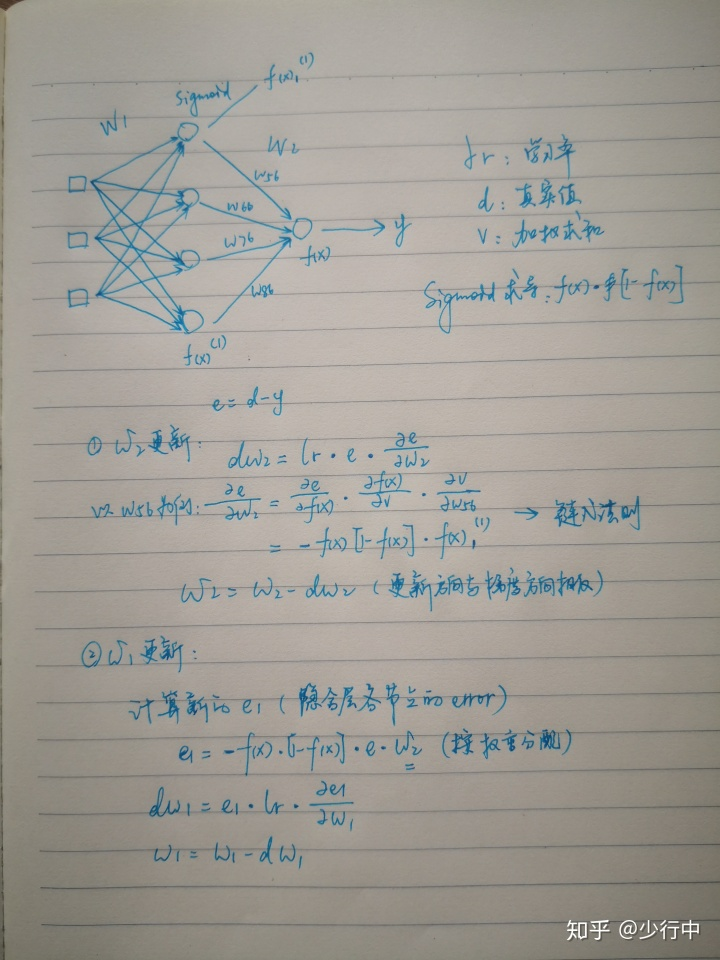下附matlab代码：1.定义sigmoid函数，方便随时调用：function y=Sigmoid(x)y=1./(1+exp(-x));end2.定义反向传播函数：function [W1,W2]=bp(W1,W2,X,Y)lr=0.9; %学习率n=4; %样本容量for k=1:n    x=X(k,:)';    y=Y(k);    v1=W1*x;    y_hat1=Sigmoid(v1);    v=W2*y_hat1;    y_hat=Sigmoid(v);    e=y-y_hat;    delta=-y_hat.*(1-y_hat).*e;    e1=W2'*delta;    delta1=y_hat1.*(1-y_hat1).*e1;    dW1=lr*delta1*x';    W1=W1-dW1;    dW2=lr*delta*y_hat1';    W2=W2-dW2;endend3.用一组数据测试一下效果：X=[0,0,1;0,1,1;1,0,1;1,1,1];Y=[0;1;1;0];W1=2*rand(4,3)-1;W2=2*rand(1,4)-1;for epoch=1:10000    [W1,W2]=bp(W1,W2,X,Y);endV1=W1*X';Y_hat1=Sigmoid(V1);V=W2*Y_hat1;Y_hat=Sigmoid(V);disp('预测值：');disp(Y_hat);error=Y'-Y_hat;disp('误差：');disp(error)效果如下，拟合效果还可以：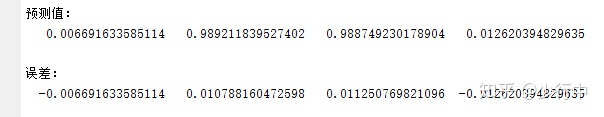以上就是matlab实现反向传播的过程，如有错误欢迎随时指出~
展开全文• 之前code都是将所有的实现放在同一个.m文件，导致程序很混乱冗长，过了几天容易忘记，便于后期修改维护，于是开始学习将程序封装。即将程序封装成函数，再调用，就比较清晰。 1、函数编写 语法： function ...
之前写code都是将所有的实现放在同一个.m文件，导致程序很混乱冗长，过了几天容易忘记，不便于后期修改维护，于是开始学习将程序封装。即将程序封装成函数，再调用，就比较清晰。

1、函数编写

语法：

function [输出参数列表] = 函数名（输入参数列表）        （注：此语句结尾没有分号）

注释部分

函数体

如：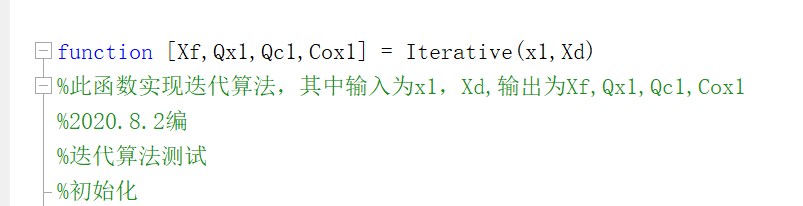将整个函数编写完后，接下来就保存.m文件，文件名为函数名.m   即上例中应保存为Iterative.m

2、调用函数

注：
1. 函数调用的时候，参数顺序应该与定义的时候一样。
2. 函数可以嵌套
3. 函数可以自己调用自己，实现递归

2.1 可直接在命令行窗口调用

如：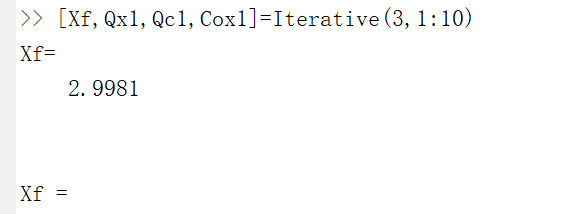2.2  在其他函数文件中调用

如：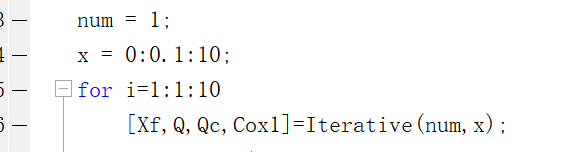3、完整参考例子

1）函数文件： power.m
function [out1, out2] = power(in1, in2, n)
%pow
out1 = (in1 + in2)^n;
out2 = (in1 - in2)^n;
2) 调用文件
a = 10;
b = 8;
for k = 0:1:10
[out1[k], out2[k]] = power(a, b, k);
end

展开全文• 汇总一下matlab 读/文本方法 读文本 1.文本只包含数字、科学记数法； testfile.txt 文本内容如下 1 2 3 4 5 6 1,2,3 4,5,6 1,2;3 1e+2 1.32e+1 2e+2 1e+2 1.32e+1 2e+2 函数：推荐使用 load 函数，代码如下，空格...
• 最近期末考试结束了，自己立下了一个flag，自学MATLAB这篇文章的目的就在于将自己所学的知识输出，希望能够帮到你。大家一起相互学习吧！话多说，下面直接进入主题。01matlab系统环境1.matlab操作界面的组成⑴...
• 一、将某MATLAB函数转换为C/C++文件输出 1.新建一个.m文件（这个文件必须是函数文件，函数名和文件名必须一致），在.m文件中把求逆函数写好，如下 2.在MATLAB软件中找到Coder 3.定义输入类型，完成之后点击Next ...
• 一、几点背景知识： 1.matlab提供了许多将影响读写...但是对tiff影像则适用，系统会出现：“无法输出single的图  像······“的报错提示。若将数据强制转换为double，则在ENVI等软件中显示为空白影像，且无法保数据
• 语法： options = optimset('param1',...%带输入或输出参数时显示完整的参数列表及其有效值。 options = optimset（带输入参数） %创建 options 结构体 options，其中所有字段设置为 []。 该函数的其他用法没.
• 定义变量a，b，c，计算输出d >> a=-3;b=2;c=5;>> d=(a^2+b)/c;>>...执行时直接在命令窗口文件名字 ...定义函数 ...保存函数，名字要一致 ...但是函数只能被调用，能直接运行 或...
• Matlab提供多种能够进行文件读写的函数，这些函数Matlab语言的一部分，并需要任何额外的工具箱的支持。 文本文件 Matlab中对文件的读写函数可以分为2大类：高级函数和低级函数。简单的说，高级函数的调用...
• 我们在使用matlab的solve这样函数时，经常遇到这样的情况，就是方程中含有一些已知参数以便加以控制，但是如何才能得到数值解？举例如下： var1=100 要解var1*x^2+20*x=0这个方程，如果直接 solve('var1*x^...
• 最近做的东西涉及到matlab中simulink工具及其内嵌函数。...回到学校发现有很大的BUG，输出结果能用（其实在家就发现了）。 在matlab function下编写.m文件，因为这个.m文件是内嵌到simulink中，他的参数不会存入ma...
• matlab中，无论是内建函数还是工具箱函数， 2很常见， 这可能是因为2英文two和to发音相同。...　函数功能： 把数值转换成字符串， 转换后可以使用fprintf或disp函数进行输出。在matlab命令窗口中键入doc int2str或h
• [图片说明](https://img-ask.csdn.net/upload/201806/02/1527937699_491662.png其中，输入u通过 fwrite(s,u)输出知道这么对不对）;而输出y=fread(s,1,'uint8')，![图片说明]...
• 1.fopen函数  该函数用于打开文件以供读写，其调用格式为：[fid,errmsg]=fopen(filename,permission)  选项说明：1）fid:函数返回值类型，为整数类型。...fid=1，标准输出文件（屏幕）。fid=2，标
• 神经网络为卷积神经网络，分别是输入层、卷积层1、激活函数、池化层1、dropoutout层（主要防止过拟合）、卷积层2、激活函数、池化层2、dropoutout层（主要防止过拟合）、全连接层和输出层。 其结构参数如下神经网络 深度学习 卷积神经网络
• 一个数据处理的程序，需要多个变量，使用eval函数，结果一直在下面输出结果，有点烦。 例如给h1,h2,h3,h4分别赋值1,2,3,4： for i = 1:4 eval(strcat('h',num2str(i),'=',num2str(i))); end 输出结果：（想屏蔽...
• 代码： function [sys,x0,str,ts] = linePt_r001(t,x,u,flag) switch flag case 0 [sys,x0,str,ts] = mdlInitializeSizes;...自定义函数不能在simulink里运行，matalbR2019b版本</p>
• ## MATLAB 正则表达式

千次阅读 2018-12-18 21:46:50
文章目录MATLAB 正则表达式与正则表达式相关的函数regexp 用法输出类型如何构建 exp元字符字符转义重复限定符重复限定符的三种模式分组运算符锚点选项（option）例子 与正则表达式相关的函数 matlab 中与正则...
• MATLAB BP神经网络中仿真结果与手工计算符合的解决办法前言...经过查阅后发现在旧版本中的newff函数，神经网络输入值的归一化以及输出值的反归一化是编程者自己的，而新版本的newff函数 MATLAB则会自动帮我们完成归神经网络
• MATLAB允许两个程序文件： 脚本 - 脚本文件 .m扩展程序文件。在这些文件中的一系列命令，想一起执行。脚本接受输入和返回任何输出。他们在工作区中的数据操作。 函数 - 函数文件.m扩展程序文件。...
• MATLAB 7.0是一个高级的矩阵/阵列语言，它包含控制语句、函数、数据结构、输入和输出和面向对象编程特点。这是我在大三上学期上图像处理的时候用到的软件，当时学得也没多好，课程结束后就再也没有用过了。第一次...
• ## MATLAB中的int2str

千次阅读 2018-08-17 17:16:49
matlab中，无论是内建函数还是工具箱函数， 2很常见， 这可能是因为2英文two和to发音相同。...函数功能： 把数值转换成字符串， 转换后可以使用fprintf或disp函数进行输出。在matlab命令窗口中键入...
• ## matlab编程小结

千次阅读 2018-01-18 23:14:18
之前学习matlab编程不够系统和全面，对很多函数不太了解。数字图像处理和矩阵的一些常用处理居然不太熟悉，停留在学习的舒适区，有点不应该。 从18年开始，保持技术博客的习惯。一个重要的转变：从转载博客到...
• MATLAB允许两种程序文件：脚本和函数。脚本用来执行一系列命令，但是接受输入和输出，只是为了方便执行某些特定的经常要重复用的命令而已。函数接受输入和输出，内部变量是本地的函数。创建并运行脚本文件可以用...
• 最近接触并学习了Matlab,充分感受到了Matlab的强大，正所谓好记忆不如烂笔头，所以在此一下学习笔记，以便后续温习。因为Matlab是一个十分重实践的软件，所以学习笔记我打算按照常规写法来进行，而是通过作者在...矩阵
• ## Matlab文件操作

千次阅读 2008-04-02 11:18:00
今天想用matlab来预处理数据，可是确太清楚matlab上的文件操作，于是上网搜索，总结如下：文件操作是一种重要的输入输出方式，即从数据文件读取数据或将结果写入数据文件。MATLAB提供了一系列低层输入输出函数，...string 磁盘 float 存储
• ## matlab的多项式基础

千次阅读 2017-02-16 20:49:23
多项式的表达可以一个关于多项式输出函数来直观的表示。 2.多项式的四则运算及导数，积分，求根–多项式的加法和减法运算就为普通两个行向量之间的运算。但是由于多项式的项数可能一样，所以需要在在最高# matlab写函数不输出matlab 订阅